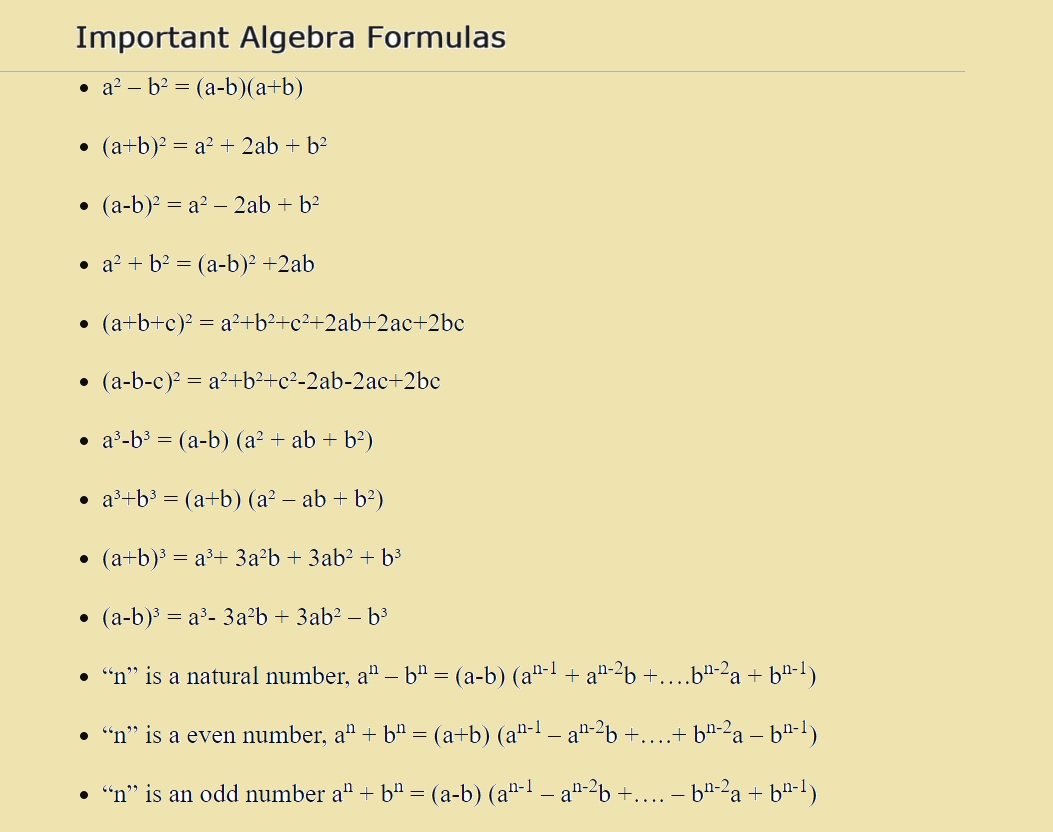# Algebra Formulas: Learn With Definitions, Formulas, and Examples

Algebra is a fundamental branch of mathematics that deals with mathematical symbols and the rules for manipulating those symbols. It is a powerful tool used to represent and solve real-world problems in various fields, from science and engineering to finance and everyday life. In this comprehensive essay, we will explore the definition of algebra, and essential algebraic formulas, and provide examples to illustrate their application.

## Definition of Algebra

Algebra is a branch of mathematics that uses symbols and letters to represent numbers and quantities in mathematical expressions, equations, and formulas. It is concerned with finding unknown values and understanding the relationships between these values.

Algebra allows us to generalize and find patterns in numbers and operations, making it a versatile tool for solving a wide range of mathematical and real-world problems.

## Basic Algebraic Concepts

1. Variables: In algebra, variables are symbols (often letters like x, y, or z) used to represent unknown or changing values. Variables are essential for writing algebraic expressions and equations.
2. Expressions: Algebraic expressions consist of variables, constants, and mathematical operations (addition, subtraction, multiplication, division). For example, 2x + 3y – 5 is an algebraic expression.
3. Equations: Equations are mathematical statements that assert two expressions are equal. They often contain an equal sign (=) and can be solved to find the values of the variables. An example is 2x + 3 = 7.

## Essential Algebraic Formulas and Examples

1. Linear Equations:
• Formula: ax + b = c
• Example: 3x + 2 = 11
• Solution: x = (11 – 2) / 3
• Solution: x = 3
• Formula: ax^2 + bx + c = 0
• Example: 2x^2 – 5x + 3 = 0
• Solution: x = (5 ± √(5^2 – 423)) / (2*2)
• Solutions: x = (5 ± √7)/4
3. Exponents:
• Formula: x^n * x^m = x^(n + m)
• Example: 2^3 * 2^2 = 2^(3 + 2)
• Simplified: 2^3 * 2^2 = 2^5
4. Polynomials:
• Formula: a_nx^n + a_(n-1)x^(n-1) + … + a_1x + a_0
• Example: 3x^2 – 2x + 5
• This is a polynomial of degree 2 (quadratic).
5. Factoring:
• Formula: a^2 – b^2 = (a + b)(a – b)
• Example: x^2 – 4
• Factored: (x + 2)(x – 2)
6. Systems of Equations:
• Formula: Multiple equations with multiple variables
• Example:
• 2x + 3y = 12
• 4x – y = 7
• Solution: x = 3, y = 2
7. Inequalities:
• Formula: x < a, x > a, x ≤ a, x ≥ a
• Example: 2x < 10
• Solution: x < 5
8. Functions:
• Formula: f(x) = 2x + 3
• Example: If f(x) = 2x + 3, find f(4).
• Solution: f(4) = 2 * 4 + 3 = 8 + 3 = 11
• Formula: x = (-b ± √(b^2 – 4ac)) / (2a)
• Example: Solve 2x^2 – 5x + 3 = 0.
• Solution: x = (5 ± √(5^2 – 4 * 2 * 3)) / (2 * 2)
• Solutions: x = (5 ± √7)/4

Algebra is a powerful mathematical tool with widespread applications in various fields. It involves the use of variables, expressions, equations, and formulas to represent, manipulate, and solve problems. The essential algebraic concepts and formulas covered in this essay are the building blocks for solving a wide range of mathematical and real-world challenges. By mastering algebra, individuals can enhance their problem-solving abilities and gain a deeper understanding of the mathematical world around them.